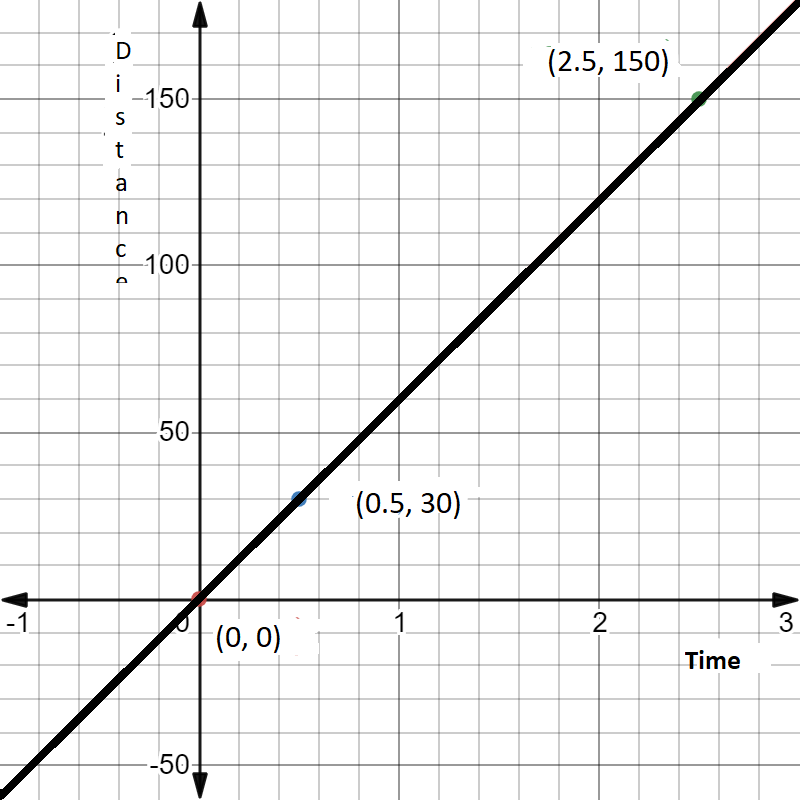# Aarushi was driving a car with uniform speed of 60 km/h. Draw distance-time graph. From the graph, find the distance travelled by Aarushi in.$2\frac{1}{2}$ hours

Given:

Aarushi was driving a car with uniform speed of 60 km/h.

To do:

We have to draw distance-time graph and find the distance travelled by Aarushi in $2\frac{1}{2}$ hours.

Solution:

Speed of the car $= 60\ km/h$.

We know that,

Speed $=\frac{\text { Distance }}{\text { time }}$

Let the distance be $y$ and time be $x$

Speed $=\frac{y}{x}$

$=60$

$\Rightarrow y=60x$

To represent the above equation graphically we need at least two solutions for the equation.

If $x=0.5$, then $y=60 \times 0.5=30$

If $x=2.5$, then $y=60 \times 2.5=150$

 $x$ $0.5$ $2.5$ $y$ $30$ $150$

The above situation can be plotted graphically as below:From the graph,

The distance travelled by Aarushi in $2\frac{1}{2}$ hours is 150 km.

Updated on: 10-Oct-2022

99 Views